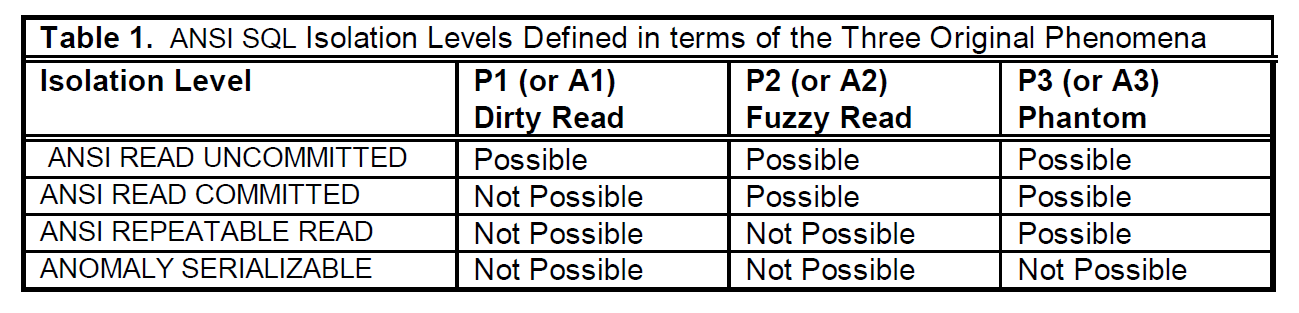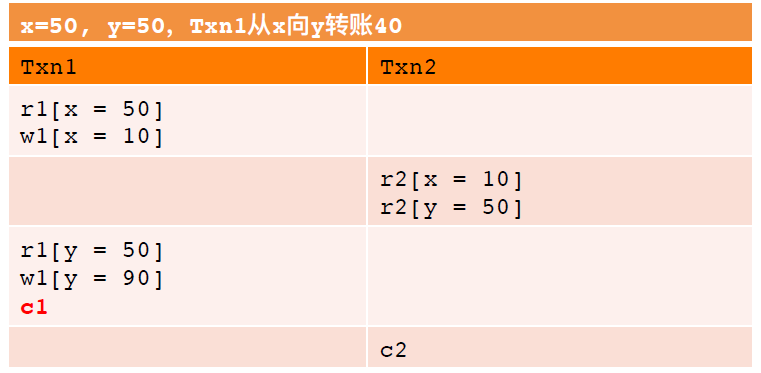事务的隔离级别

# 事务的隔离级别

2020-09-03

## 1.事务隔离级别

Dirty read: SQL-transaction T1 modifies a row. SQL- transaction T2 then reads that row before T1 performs a COMMIT. If T1 then performs a ROLLBACK, T2 will have read a row that was never committed and that may thus be considered to have never existed.
Non-repeatable read: SQL-transaction T1 reads a row. SQL- transaction T2 then modifies or deletes that row and performs a COMMIT. If T1 then attempts to reread the row, it may receive the modified value or discover that the row has been deleted.
Phantom: SQL-transaction T1 reads the set of rows N that satisfy some . SQL-transaction T2 then executes SQL-statements that generate one or more rows that satisfy the used by SQL-transaction T1. If SQL-transaction T1 then repeats the initial read with the same , it obtains a different collection of rows.

A1 Dirty Read：w1[x] ... r2[x] ... (a1 and c2 in any order)
A2 Fuzzy Read：r1[x] ... w2[x] ... c2 ... r1[x] ... c1
A3 Phantom Read：r1[P] ... w2[y in P] ... c2 ... r1[P] ... c1P1 Dirty Read: w1[x]...r2[x]...(c1 or a1)
P2 Fuzzy Read: r1[x]...w2[x]...(c1 or a1)
P3 Phantom: r1[P]...w2[y in P]...(c1 or a1)

P4 Lost Update：r1[x]...w2[x]...w1[x]...c1
A5A Read Skew：r1[x]…w2[x]... w2[y]…c2…r1[y] …(c1 or a1)
A5B Write Skew：r1[x]…r2[y]…w1[y]…w2[x]…(c1 and c2 occur)
A5B2 Write Skew2：r1[P]... r2[P]…w1[y in P]…w2[x in P]...(c1 and c2 occur)

r1[x=50] r2[x=50] w2[x=60] c2 w1[x=70] c1

Lost Update:事务1和事务2同时向同一个账户x分别充20和10块，事务1后提交，将70块写入数据库，事务2提交结果60块被覆盖。正确的情况下，事务1和2提交成功，账户里应该有80块。

(x+y=100) r1[x=50] w2[x=10] w2[y=90] c2 r1[y=90] c1

(x+y>=60) r1[x=50] r2[y=50] w1[y=10] c1 w2[x=10] c2

Write Skew：x和y账户分别有50块钱，加起来共100块。假设存在某种约束，x和y账户的钱加起来不得少于60块。事务1和事务2在自认为不破坏约束的情况下（分别读了x账户和y账户），再分别从y账户和x账户取走40。但事实上，这两个事务完成后，x+y=20，约束条件被破坏。

(count(P)<=4):r1[count(P)=3],r2[count(P)=3],insert1[x in P],insert2[y in P],c1,c2,

Write Skew2：将Write Skew的条件改为范围。